Function Repository Resource:

# RandomSplit

Randomly divide a list into a specified number of segments

Contributed by: Aster Ctor
 ResourceFunction["RandomSplit"][list,n] randomly cuts list into n segments. ResourceFunction["RandomSplit"][n] represents an operator form of ResourceFunction["RandomSplit"] that can be applied to an expression.

## Examples

### Basic Examples (2)

Randomly cut a list of length 10 into five segments:

 In:=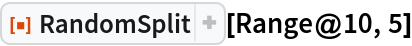Out=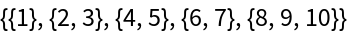Use another random cut:

 In:=Out=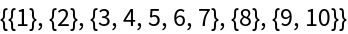### Scope (2)

Use the operator form:

 In:=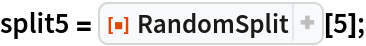In:=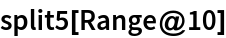Out=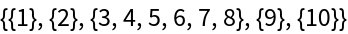The operator form is still random in each call:

 In:=Out=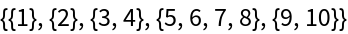### Possible Issues (1)

If the number of segments exceeds the total length of the list, a message is issued:

 In:=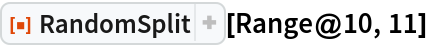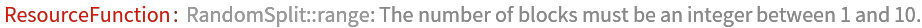## Publisher

Aster Ctor (MoeNet)

## Version History

• 1.0.0 – 02 January 2020